# hic3defdr¶A genome-scale differential loop finder.

For the latest source, discussion, etc, please visit the Bitbucket repository at https://bitbucket.org/creminslab/hic3defdr

This is the repository for hic3defdr, a Python package that provides an implementation of the 3DeFDR-HiC differential loop calling method proposed in a manuscript currently under review.

## Installation¶

We require Python 2.7.11+ or 3.6+ and the dependencies listed in `setup.py`.

A typical quick install process should be:

```\$ virtualenv venv
\$ source venv/bin/activate
(venv)\$ pip install hic3defdr
```

A typical dev-mode install process should be:

```\$ git clone https://bitbucket.org/creminslab/hic3defdr.git
\$ cd hic3defdr
\$ virtualenv venv
\$ source venv/bin/activate
(venv)\$ pip install -e .
```

If installation succeeded then `hic3defdr.HiC3DeFDR` should be importable from an interactive shell started in some other directory:

```(venv)\$ cd <some other directory>
(venv)\$ python
>>> from hic3defdr import HiC3DeFDR
```

### Optional dependencies¶

Evaluating simulations requires scikit-learn:

```(venv)\$ pip install scikit-learn
```

To display progress bars during selected steps of the analysis, install tqdm:

```(venv)\$ pip install tqdm
```

## Basic walkthrough¶

Before we start, we’ll seed numpy’s random number generator for reproducibility:

```>>> import numpy as np
>>> np.random.seed(42)
```

To analyze the ES_1, ES_3, NPC_1, and NPC_2 reps of the dataset dataset from Bonev et al. 2017 with default parameters, we would first describe the dataset in terms of replicate names, chromosome names, and a design matrix. We will just analyze chromosomes 18 and 19 here for illustrative purposes.

```>>> import pandas as pd
>>>
>>> repnames = ['ES_1', 'ES_3', 'NPC_1', 'NPC_2']
>>> #chroms = ['chr%i' % i for i in range(1, 20)] + ['chrX']
>>> chroms = ['chr18', 'chr19']
>>> design = pd.DataFrame({'ES': [1, 1, 0, 0], 'NPC': [0, 0, 1, 1]},
...                       dtype=bool, index=repnames)
```

```\$ python -m hic3defdr.util.demo_data
```

or from inside an interactive shell:

```>>> from hic3defdr.util.demo_data import ensure_demo_data
>>> ensure_demo_data()
```

The data will be downloaded to a folder called `hic3defdr-demo-data` under your home directory.

The required input files consist of:

• upper triangular, raw contact matrices in `scipy.sparse` NPZ format,
• bias vectors in plain-text `np.savetxt()` format, and
• loop cluster files in sparse JSON format (see below for more details), specifying the locations of loops present in each condition

TODO: explain how to import data from common Hi-C analysis tools into this format

We would next describe the location of the input data files and use those to construct a `HiC3DeFDR` object:

```>>> import os.path
>>> from hic3defdr import HiC3DeFDR
>>>
>>> base_path = os.path.expanduser('~/hic3defdr-demo-data/')
>>> h = HiC3DeFDR(
...     raw_npz_patterns=[base_path + '<rep>/<chrom>_raw.npz'.replace('<rep>', repname) for repname in repnames],
...     bias_patterns=[base_path + '<rep>/<chrom>_kr.bias'.replace('<rep>', repname) for repname in repnames],
...     chroms=chroms,
...     design=design,
...     outdir='output',
...     loop_patterns={c: base_path + 'clusters/%s_<chrom>_clusters.json' % c for c in ['ES', 'NPC']},
...     res=10000
... )
creating directory output
```

This object saves itself to disk, so it can be re-loaded at any time:

```>>> h = HiC3DeFDR.load('output')
```

To run the analysis for all chromosomes through q-values, run:

```>>> h.run_to_qvalues()
```

To threshold, cluster, and classify the significantly differential loops, and collect all the results into a single TSV output file, run:

```>>> h.collect()
```

The output file will be written to `output/results_0.01_3.tsv`, where “output” refers to the `outdir` we passed when constructing the `HiC3DeFDR` object, “0.01” refers to the default FDR of 1%, and “3” refers to the default cluster size threshold of 3.

```>>> import pandas as pd
us_chrom  ...  classification
loop_id                                               ...
chr18:3480000-3500000_chr18:4680000-4710000    chr18  ...    constitutive
chr18:3490000-3510000_chr18:3790000-3810000    chr18  ...              ES
chr18:3490000-3510000_chr18:3970000-3990000    chr18  ...    constitutive
chr18:3490000-3510000_chr18:4170000-4200000    chr18  ...    constitutive
chr18:3490000-3520000_chr18:4120000-4150000    chr18  ...    constitutive

[5 rows x 9 columns]
```

See the section “TSV output format” below for more details about the output format.

## Step-by-step walkthrough¶

Calling `h.run_to_qvalues()` runs the four main steps of the analysis in sequence. These four steps are described in further detail below. Any kwargs passed to `h.run_to_qvalues()` will be passed along to the appropriate step; see `help(HiC3DeFDR.run_to_qvalues)` for details.

### Step 1: Preparing input data¶

The function call `h.prepare_data()` prepares the input raw contact matrices and bias vectors specified by `h.raw_npz_patterns` and `h.bias_patterns` for all chromosomes specified in `h.chroms`, performs library size normalization, and determines what points should be considered for dispersion estimation. This creates intermediate files in the output directory `h.outdir` that represent the raw and scaled values, as well as the estimated size factors, and a boolean vector `disp_idx` indicating which points will be used for dispersion estimation. If `loop_patterns` was passed to the constructor, an additional boolean vector `loop_idx` is created to mark which points that pass `disp_idx` lie within looping interactions specified by `h.loop_patterns`. The raw and scaled data are stored in a rectangular matrix format where each row is a pixel of the contact matrix and each column is a replicate. If the size factors are estimated as a function of distance, the estimated size factors are also stored in this format. Two separate vectors called `row` and `col` are used to store the row and column index of the pixel represented by each row of the rectangular matrices. Together, the `row` and `col` vectors plus any of the rectangular matrices represent a generalization of a COO format sparse matrix to multiple replicates (in the standard COO format the `row` and `col` vectors are complemented by a single `value` vector).

The size factors can be estimated with a variety of methods defined in the `hic3defdr.scaling` module. The method to use is specified by the `norm` kwarg passed to `h.prepare_data()`. Some of these methods estimate size factors as a function of interaction distance, instead of simply estimating one size factor for each replicate as is common in RNA-seq differential expression analysis. When these methods are used, the number of bins to use when binning distances for distance-based estimation of the size factors can be specified with the `n_bins` kwarg. The defaults for this function use the conditional median of ratios approach (`hic3defdr.scaling.conditional_mor`) and an automatically-selected number of bins.

### Step 2: Estimating dispersions¶

The function call `h.estimate_disp()` estimates the dispersion parameters at each distance scale in the data and fits a lowess curve through the graph of distance versus dispersion to obtain final smoothed dispersion estimates for each pixel.

The `estimator` kwarg on `h.estimate_disp()` specifies which dispersion estimation method to use, out of a selection of options defined in the `hic3defdr.dispersion` module. The default is to use quantile-adjusted conditional maximum likelihood (qCML).

### Step 3: Likelihood ratio test¶

The function call `h.lrt()` performs a likelihood ratio test (LRT) for each pixel. This LRT compares a null model of no differential expression (fitting one true mean parameter shared by all replicates irrespective of condition) to an alternative model in which the two conditions have different true mean parameters.

### Step 4: False discovery rate (FDR) control¶

The function call `h.bh()` performs Benjamini-Hochberg FDR correction on the p-values called via the LRT in the previous step, considering only a subset of pixels that are involved in looping interactions (as specified by `h.loop_patterns`; if `loop_patterns` was not passed to the constructor then all pixels are included in the BH-FDR correction). This results in final q-values for each loop pixel.

### Thresholding, clustering, and classification¶

We can threshold, cluster, classify, and collect the significantly differential loops:

```>>> h.collect(fdr=0.05, cluster_size=3)
```

We can also sweep across FDR and/or cluster size thresholds:

```>>> h.collect(fdr=[0.01, 0.05], cluster_size=[3, 4])
```

The final output file for each combination of thresholding parameters will be written to `<h.outdir>/results_<fdr>_<cluster_size>.tsv`.

This example walkthrough should take less than 5 minutes for the two chromosomes included in the demo data. Run time for a whole-genome analysis will depend on parallelization as discussed in the next section.

## Parallelization¶

The functions `run_to_qvalues()`, `prepare_data()`, `lrt()`, `threshold()`, `classify()`, and `simulate()` operate in a per-chromosome manner. By default, each chromosome in the dataset will be processed in series. If multiple cores and sufficient memory are available, you can use the `n_threads` kwarg on these functions to use multiple subprocesses to process multiple chromosomes in parallel. Pass either a desired number of subprocesses to use, or pass `n_threads=-1` to use all available cores. The output logged to stderr will be truncated to reduce clutter from multiple subprocesses printing to stderr at the same time. This truncation is controlled by the `verbose` kwarg which is available on some of these parallelized functions.

The function `estimate_disp()` also accepts an `n_threads` kwarg, using it to parallelize itself across distance scales.

The function `run_to_qvalues()` passes the `n_threads` kwarg through to all the steps it calls.

## Intermediates and final output files¶

All intermediates used in the computation will be saved to the disk inside the `outdir` folder as `<intermediate>_<chrom>.npy` (most intermediates), `<intermediate>_<chrom>.json`/`<intermediate>_<chrom>.tsv` (thresholded or classified clusters).

Two intermediates are not generated per chromosome. These are `disp_fn_<c>.pickle` (dispersion function estimated across all chromosomes for condition `<c>`) and `results_<f>_<s>.tsv` (final combined results from all chromosomes).

Step Intermediate Shape Description
`prepare_data()` `row` `(n_pixels,)` Top-level row index
`prepare_data()` `col` `(n_pixels,)` Top-level column index
`prepare_data()` `bias` `(n_bins, n_reps)` Bias vectors
`prepare_data()` `raw` `(n_pixels, n_reps)` Raw count values
`prepare_data()` `size_factors` `(n_reps,)` or `(n_pixels, n_reps)` Size factors
`prepare_data()` `scaled` `(n_pixels, n_reps)` Normalized count values
`prepare_data()` `disp_idx` `(n_pixels,)` Marks pixels for which dispersion is fitted
`prepare_data()` `loop_idx` `(disp_idx.sum(),)` Marks pixels which lie in loops
`estimate_disp()` `cov_per_bin` `(n_bins, n_conds)` Average mean count or distance in each bin
`estimate_disp()` `disp_per_bin` `(n_bins, n_conds)` Pooled dispersion estimates in each bin
`estimate_disp()` `disp_fn_<c>` pickled function Fitted dispersion function
`estimate_disp()` `disp` `(disp_idx.sum(), n_conds)` Smoothed dispersion estimates
`lrt()` `mu_hat_null` `(disp_idx.sum(),)` Null model mean parameters
`lrt()` `mu_hat_alt` `(disp_idx.sum(), n_conds)` Alternative model mean parameters
`lrt()` `llr` `(disp_idx.sum(),)` Log-likelihood ratio
`lrt()` `pvalues` `(disp_idx.sum(),)` LRT-based p-value
`bh()` `qvalues` `(loop_idx.sum(),)` BH-corrected q-values
`threshold()` `sig_<f>_<s>` JSON/TSV Significantly differential clusters
`threshold()` `insig_<f>_<s>` JSON/TSV Constitutive clusters
`classify()` `<c>_<f>_<s>` JSON/TSV Classified differential clusters
`collect()` `results_<f>_<s>` TSV Final results table

The table uses these abbreviations to refer to variable parts of certain intermediate names:

• `<f>`: FDR threshold
• `<s>`: cluster size threshold
• `<c>`: condition/class label

## Sparse JSON cluster format¶

This is the format used both for supplying pre-identified, per-condition loop clusters as input to HiC3DeFDR as well as the format in which differential and constitutive interaction clusters are reported as output.

The format describes the clusters on each chromosome in a separate JSON file. This JSON file contains a single JSON object, which is a list of list of list of integers. The inner lists are all of length 2 and represent specific pixels of the heatmap for that chromosome in terms of there row and column coordinates in zero-indexed bin units. The outer middle lists can be of any length and represent groups of pixels that belong to the same cluster. The length of the outer list corresponds to the number of clusters on that chromosome.

These clusters can be loaded into and written from the corresponding plain Python objects using `hic3defdr.clusters.load_clusters()` and `hic3defdr.clusters.save_clusters()`, respectively. The plain Python objects can be converted to and from scipy sparse matrix objects using `hic3defdr.clusters.clusters_to_coo()` and `hic3defdr.clusters.convert_cluster_array_to_sparse()`, respectively.

## TSV output format¶

The final TSV output file `results_<f>_<s>.tsv` has as its first column a `loop_id`, a string of the form `<us_chrom>:<us_start>-<us_end>_<ds_chrom>:<ds_start>-<ds_end>` specifying a rectangle that surrounds the cluster of pixels that make up the loop. The next six columns are the six individual pieces of this `loop_id`. This is followed by:

• `cluster_size`: the number of pixels in the cluster
• `cluster`: a list of the exact (row, col) indices of the pixels in the cluster
• `classification`: this will be “constitutive” if the loop is not differential, or the name of the condition the loop is specific to (i.e., strongest in) if it is differential

## Visualizations¶

The `HiC3DeFDR` object can be used to draw visualizations of the analysis.

The visualization functions are wrapped with the @plotter decorator and therefore all support the convenience kwargs provided by that decorator (such as `outfile`).

### Distance dependence curves before and after scaling¶

```>>> _ = h.plot_dd_curves('chr18', outfile='images/dd.png')
```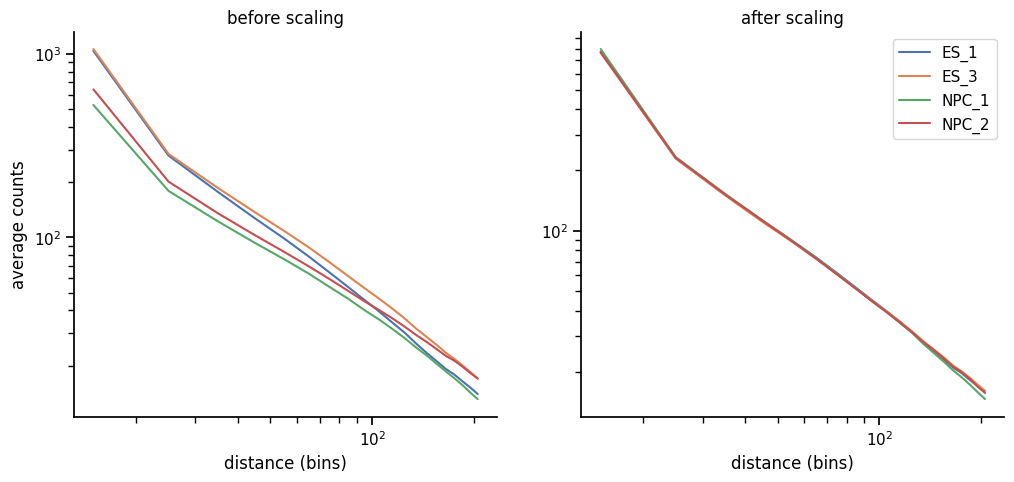### Simple heatmap plotting¶

```>>> _ = h.plot_heatmap('chr18', slice(1000, 1100), slice(1000, 1100), rep='ES_1',
...                    outfile='images/heatmap.png')
```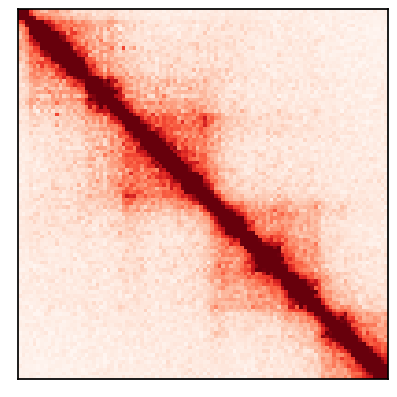By default, this plots data at the “scaled” stage (normalized for bias and sequencing depth differences), but you can plot any stage of the data by passing a `stage` kwarg.

### Condition-average heatmap plotting¶

To plot a within-condition average heatmap, pass a `stage` name with a ‘_mean’ suffix appended and `cond` to specify the condition to average within:

```>>> _ = h.plot_heatmap('chr18', slice(1000, 1100), slice(1000, 1100),
...                    stage='scaled_mean', cond='ES',
...                    outfile='images/heatmap_mean.png')
```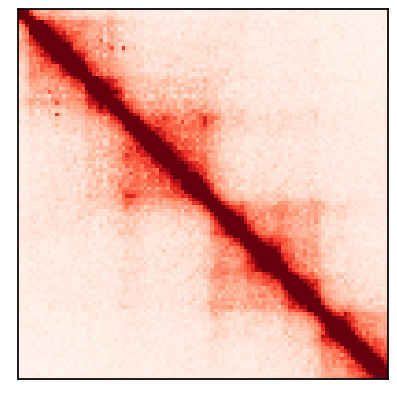### Loop zoomin heatmap plotting¶

We can combine `h.plot_heatmap()` with the `load_clusters()` and `cluster_to_slices()` utility functions in `hic3defdr.util.clusters` to plot zoomins around specific loops:

```>>> from hic3defdr.util.clusters import load_clusters, cluster_to_slices
>>> chrom = 'chr18'
>>> slices = cluster_to_slices(clusters)
>>> _ = h.plot_heatmap(chrom, *slices, rep='ES_1', outfile='images/zoomin.png')
```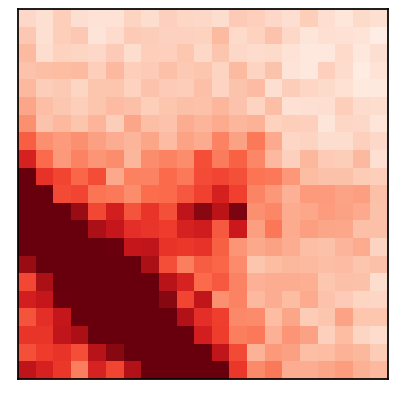### Per-pixel significance plotting¶

We can pass `stage='qvalues'` to `h.plot_heatmap()` to draw heatmaps showing the significance of each pixel:

```>>> _ = h.plot_heatmap('chr18', slice(1310, 1370), slice(1310, 1370),
...                    stage='qvalues', cmap='bwr_r', vmin=0.099, vmax=0.101,
...                    outfile='images/heatmap_sig.png')
```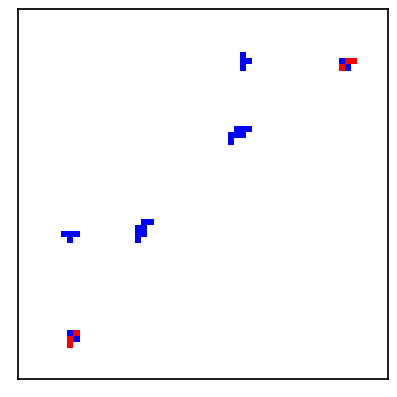By passing `cmap='bwr_r'` we ensure that significant, low q-value pixels will be red while insignificant, high q-value pixels will be blue. By passing `vmin=0.099, vmax=0.101`, we ensure that the colorbar is focused on a narrow range around an FDR threshold of 10%, allowing us to more easily tell the difference between significant and insignificant pixels.

### Correlation matrices¶

```>>> _ = h.plot_correlation_matrix(outfile='images/correlation.png')
```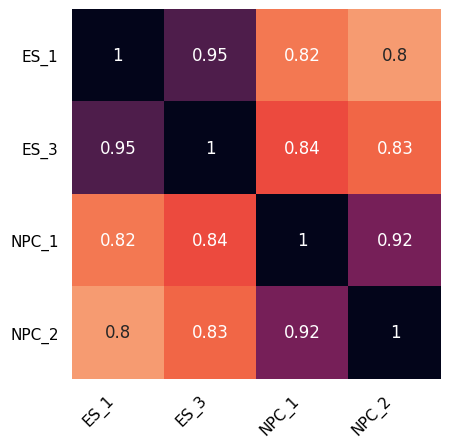### Dispersion fitting¶

```>>> _ = h.plot_dispersion_fit('ES', outfile='images/ddr.png')
```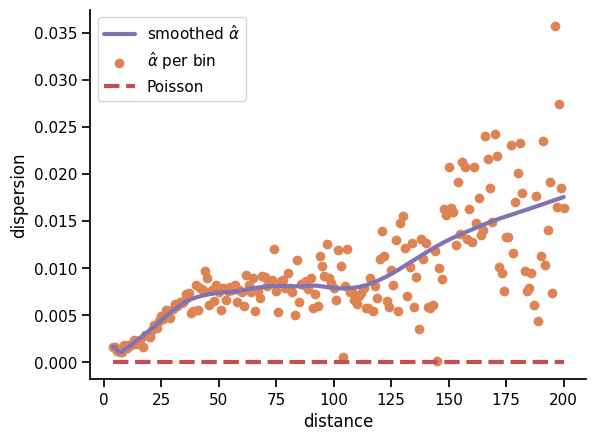It’s possible to use the the one-dimensional distance dependence curve to convert distances to means. Doing so allows plotting the y-axis in terms of variance. You can do this by passing `yaxis='var'`:

```>>> _ = h.plot_dispersion_fit('ES', yaxis='var', outfile='images/dvr.png')
```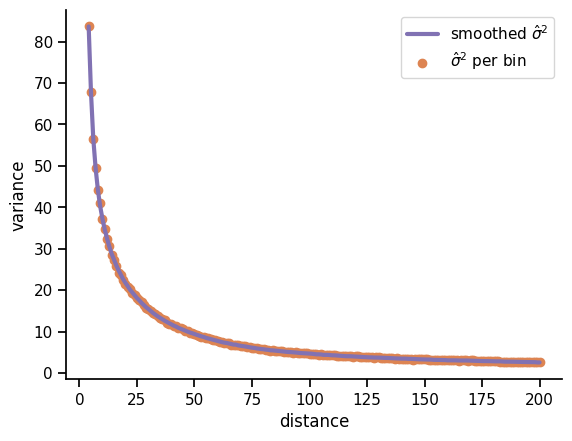Using the same trick, you can plot the x-axis in terms of mean by passing `xaxis='mean'`:

```>>> _ = h.plot_dispersion_fit('ES', xaxis='mean', yaxis='var',
...                           outfile='images/mvr.png')
```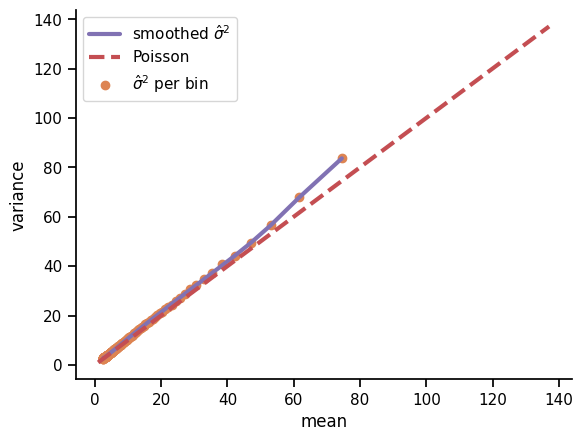At low mean and high distance, the distance dependence curve flattens out and the data become more noisy, making this conversion unreliable.

It’s also possible to show the dispersion fitted at just one distance scale, overlaying the sample mean and sample variance across replicates for each pixel as a blue hexbin plot:

```>>> _ = h.plot_dispersion_fit('ES', distance=25, hexbin=True, xaxis='mean',
...                           yaxis='var', logx=True, logy=True,
...                           outfile='images/mvr_25.png')
```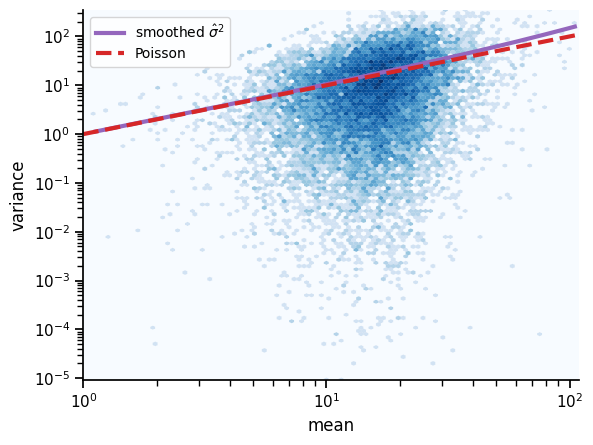If dispersion was fitted against distance rather than mean, pass `xaxis='dist'` to plot dispersion/variance versus distance.

### Comparing dispersion fits¶

It’s possible to compare different dispersion fits using the function `compare_disp_fits()` as shown here:

```>>> from hic3defdr import compare_disp_fits
>>>
>>> _ = compare_disp_fits(
...     [h.load_disp_fn(cond) for cond in h.design.columns],
...     h.design.columns,
...     max_dist=100,
...     outfile='images/disp_comparison.png'
... )
```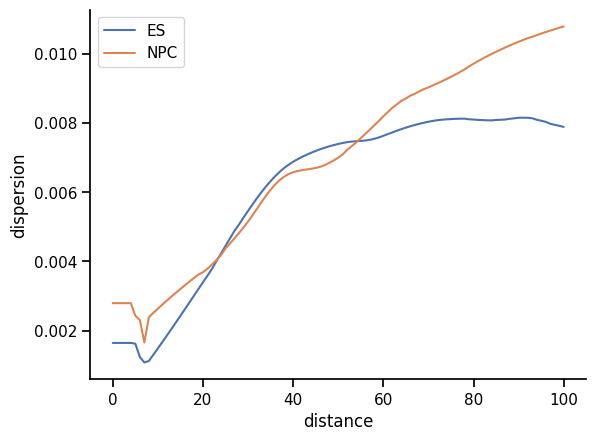### P-value distribution¶

```>>> _ = h.plot_pvalue_distribution(outfile='images/pvalue_dist.png')
```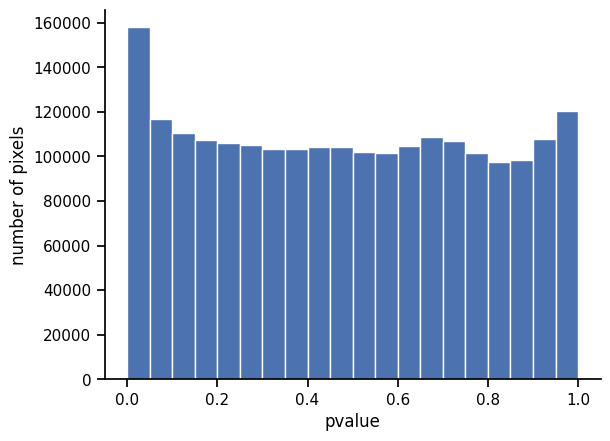By default, this plots the p-value distribution over all pixels for which dispersion was estimated. To plot the p-value distribution only over points in loops, pass `idx='loop'`.

### Q-value distribution¶

```>>> _ = h.plot_qvalue_distribution(outfile='images/qvalue_dist.png')
```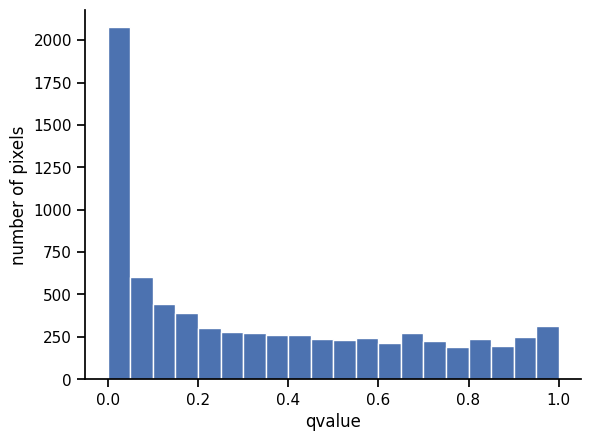### MA plot¶

```>>> _ = h.plot_ma(outfile='images/ma.png')
```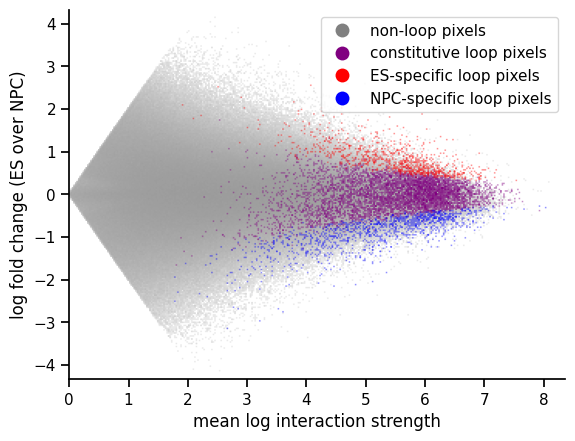### Pixel detail grid¶

```>>> _ = h.plot_grid('chr18', 2218, 2236, 20, outfile='images/grid.png')
```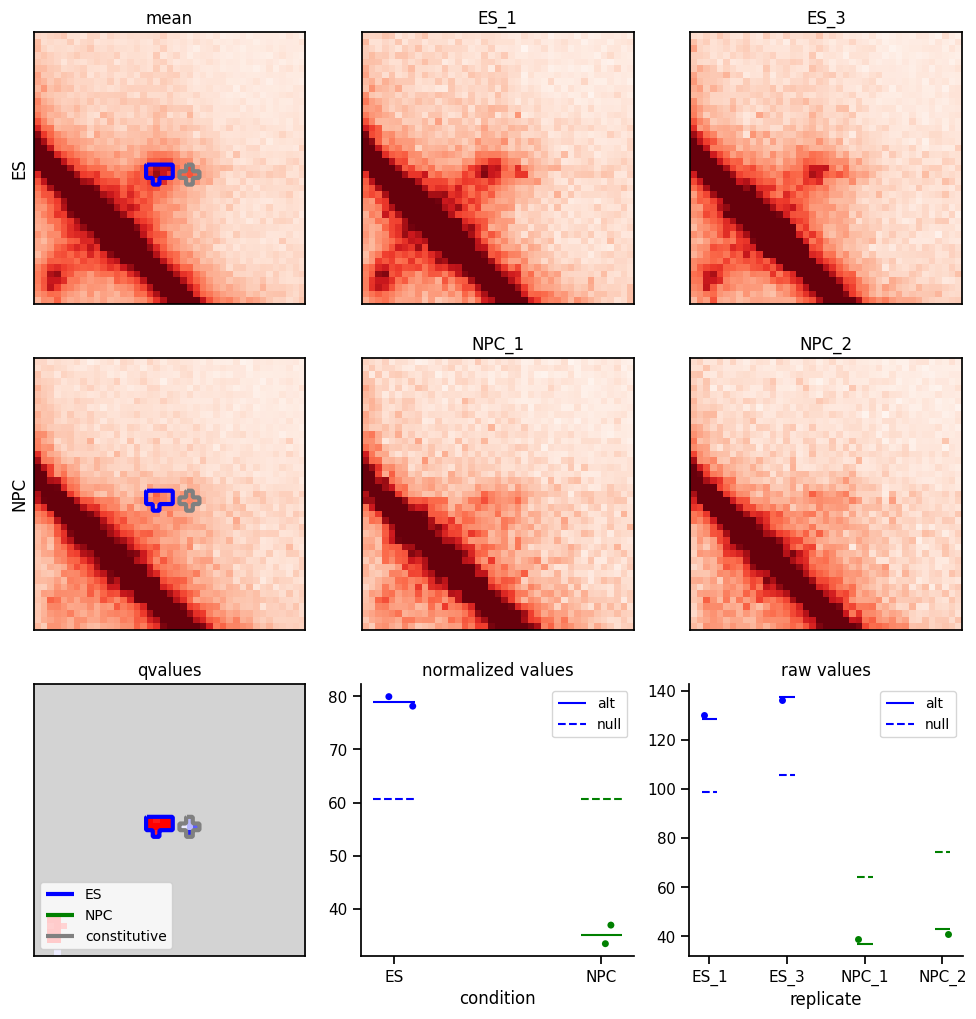The upper right heatmaps show the balanced and scaled values in each replicate, with each condition on its own row.

The upper left heatmaps show the alternative model mean parameter estimates for each condition. Significantly differential clusters are purple while constitutive ones are gray.

The lower left heatmap shows the q-values. Significantly differential clusters are orange while constitutive ones are gray.

The stripplots in the lower left show details information about the specific pixel in the center of the heatmaps (in this example `(2218, 2236)`). The dots show the values at that pixel for each replicate in normalized and raw space, repsectively. The solid and dashed lines represent the mean parameters under the alt and null models, repsectively.

Green points in the heatmaps represent points that have been filtered out. For the per-replicate heatmaps in the upper right of the grid, the only filters applied are the zero filter, bias filter, and distance filter. For the alt model mean heatmaps in the upper left, this additionally includes the dispersion filter. For the q-value heatmap in the lower left, it additionally includes the loop filter if loop locations were supplied.

### Interactive thresholding¶

In a Jupyter notebook environment with `ipywidgets` installed, you can play with thresholds on a live-updating plot by running:

```%matplotlib notebook

from ipywidgets import interact
from hic3defdr import HiC3DeFDR

_, _, outline_clusters = h.plot_grid('chr18', 2218, 2236, 50)
_ = interact(outline_clusters, fdr=[0.01, 0.05, 0.1, 0.2],
cluster_size=[3, 4])
```

## Simulation¶

After the `estimate_disp()` step has been run, a HiC3DeFDR object can be used to generate simulations of differential looping.

### Generating simulations¶

To create an ES-based simulation over all chromosomes listed in `h.chroms`, we run

```>>> from hic3defdr import HiC3DeFDR
>>>
>>> h.simulate('ES')
```

If we passed `trend='dist'` to `h.estimate_disp()`, we need to pass it to `h.simulate()` as well to ensure that the simulation function knows to treat the previously-fitted dispersion function as a function of distance.

This takes the mean of the real scaled data across the ES replicates and perturbs the loops specified in `h.loop_patterns['ES']` up or down at random to generate two new conditions called “A” and “B”. The scaled mean matrices for these conditions are then biased and scaled by the bias vectors and size factors taken from the real experimental replicates, and the ES dispersion function fitted to the real ES data is applied to the biased and scaled means to obtain dispersion values. These means and dispersions are used to draw an NB random variable for each pixel of each simulated replicate. The number of replicates in each of the simulated conditions “A” and “B” will match the design of the real analysis.

The simulated raw contact matrices will be written to disk in CSR format as `<cond><rep>_<chrom>_raw.npz` where `<cond>` is “A” or “B” and `<rep>` is the rep number within the condition. The design matrix will also be written to disk as `design.csv`.

The true labels used to perturb the loops will also be written to disk as `labels_<chrom>.txt`. This file contains as many lines as there are clusters in `h.loop_patterns['ES']`, with the `i`th line providing the label for the `i`th cluster. This file can be loaded with `np.loadtxt(..., dtype='|S7')`.

### Evaluating simulations¶

After generating simulated data, HiC3DeFDR can be run on the simulated data. Then, the true labels can be used to evaluate the performance of HiC3DeFDR on the simulated data.

Evaluation of simulated data requires scikit-learn. To install this package, run

```(venv)\$ pip install scikit-learn
```

In order to run HiC3DeFDR on the simulated data, we first need to balance the simulated raw contact matrices to obtain bias vectors for each simulated replicate and chromosome. We will assume are saved next to the raw contact matrices and named `<rep>_<chrom>_kr.bias`. One example of how this can be done is shown in the following script:

```>>> import sys
>>>
>>> import numpy as np
>>> import scipy.sparse as sparse
>>>
>>> from hic3defdr.util.filtering import filter_sparse_rows_count
>>> from hic3defdr.util.balancing import kr_balance
>>> from hic3defdr.util.printing import eprint
>>>
>>>
>>> infile_pattern = 'sim/<rep>_<chrom>_raw.npz'
>>> repnames = ['A1', 'A2', 'B1', 'B2']
>>> chroms = ['chr18', 'chr19']
>>>
>>> for repname in repnames:
...     for chrom in chroms:
...         eprint('balancing rep %s chrom %s' % (repname, chrom))
...         infile = infile_pattern.replace('<rep>', repname)\
...             .replace('<chrom>', chrom)
...         outfile = infile.replace('_raw.npz', '_kr.bias')
...         _, bias, _ = kr_balance(
...         np.savetxt(outfile, bias)
```

Next, we create a new HiC3DeFDR object to analyze the simulated data and run the analysis through to q-values:

```>>> import os.path
>>> from hic3defdr import HiC3DeFDR
>>>
>>> repnames = ['A1', 'A2', 'B1', 'B2']
>>> chroms = ['chr18', 'chr19']
>>> sim_path = 'sim/'
>>> base_path = os.path.expanduser('~/hic3defdr-demo-data/')
>>> h_sim = HiC3DeFDR(
...     raw_npz_patterns=[sim_path + '<rep>_<chrom>_raw.npz'.replace('<rep>', repname) for repname in repnames],
...     bias_patterns=[sim_path + '<rep>_<chrom>_kr.bias'.replace('<rep>', repname) for repname in repnames],
...     chroms=chroms,
...     design=sim_path + 'design.csv',
...     outdir='output-sim',
...     loop_patterns={'ES': base_path + 'clusters/ES_<chrom>_clusters.json'}
... )
creating directory output-sim
>>> h_sim.run_to_qvalues()
```

Next, we can evaluate the simulation against the clusters in `h_sim.loop_patterns['ES']` with true labels from `sim/labels_<chrom>.txt`:

```>>> h_sim.evaluate('ES', 'sim/labels_<chrom>.txt')
```

This writes a file in `h_sim`’s output directory called `eval.npz`. This file can be loaded with `np.load()` and has four keys whose values are all one dimensional vectors:

• `'thresh'`: the thresholds (in `1 - qvalue` space) which make up the convex edge of the ROC curve; all other vectors are parallel to this one
• `'fdr'`: the observed false discovery rate at each threshold
• `'tpr'`: the observed true positive rate at each threshold
• `'fpr'`: the observed false positive rate at each threshold

`eval.npz` files (possibly across many runs) can be visualized as ROC curves and FDR control curves by running:

```>>> import numpy as np
>>> from hic3defdr import plot_roc, plot_fdr
>>>
>>> _ = plot_roc([np.load('output-sim/eval.npz')], ['hic3defdr'], outfile='images/roc.png')
>>> _ = plot_fdr([np.load('output-sim/eval.npz')], ['hic3defdr'], outfile='images/fdr.png')
```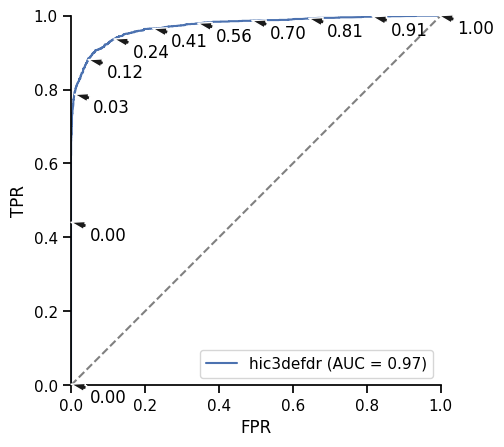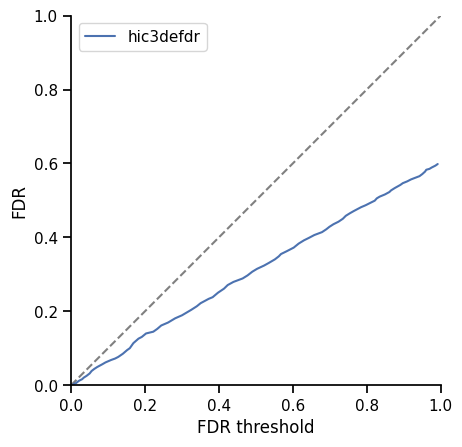Multiple `eval.npz` files can be compared in the same plot by simply adding elements to the lists in these function calls.

The ROC plot shows FPR versus TPR, with the gray diagonal line representing the performance of random guessing. The AUROC for each curve is shown in the legend. If only one curve is plotted, selected thresholds (in units of FDR threshold) are annotated with black arrows.

The FDR control plot shows the observed FDR as a function of the FDR threshold. Points below the gray diagonal line represent points at which FDR is successfully controlled.

As an added bonus, it’s also possible to evaluate the performance on specific subsets of distance scales by using the `min_dist` and `max_dist` kwargs on `HiC3DeFDR.evaluate()` as illustrated below:

```>>> dist_bins = [
...    ('short', (None, 15)),  # distances in bin units
...    ('mid', (16, 30)),
...    ('long', (31, None))
... ]
>>> for _, (min_dist, max_dist) in dist_bins:
...     h_sim.evaluate('ES', 'sim/labels_<chrom>.txt', min_dist=min_dist,
...                    max_dist=max_dist)
>>> _ = plot_roc([np.load('output-sim/eval_%s_%s.npz' % (min_dist, max_dist))
...               for _, (min_dist, max_dist) in dist_bins],
...              [label for label, _ in dist_bins],
...              outfile='images/roc_by_dist.png')
>>> _ = plot_fdr([np.load('output-sim/eval_%s_%s.npz' % (min_dist, max_dist))
...               for _, (min_dist, max_dist) in dist_bins],
...              [label for label, _ in dist_bins],
...              outfile='images/fdr_by_dist.png')
```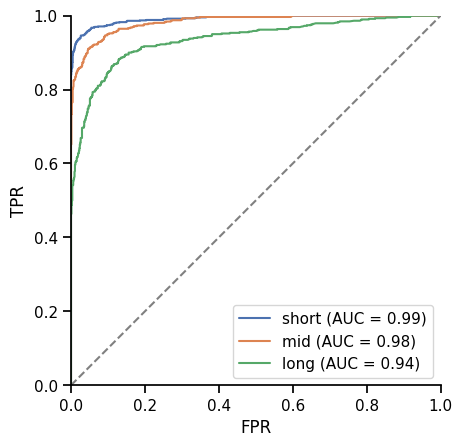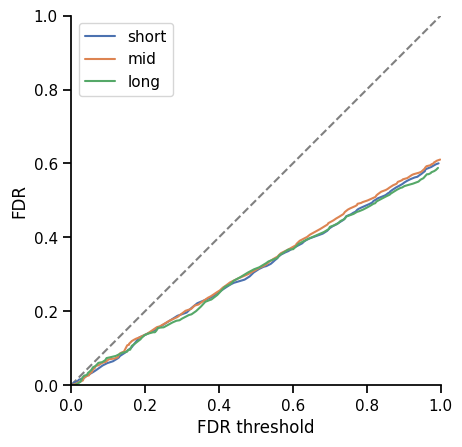It’s also possible to compare the FPR and FNR at the different subsets:

```>>> from hic3defdr import plot_fn_vs_fp
>>>
>>> _ = plot_fn_vs_fp([np.load('output-sim/eval_%s_%s.npz' % (min_dist, max_dist))
...                    for _, (min_dist, max_dist) in dist_bins],
...                   [label for label, _ in dist_bins], xlabel='distance subset',
...                   outfile='images/fn_vs_fp.png')
```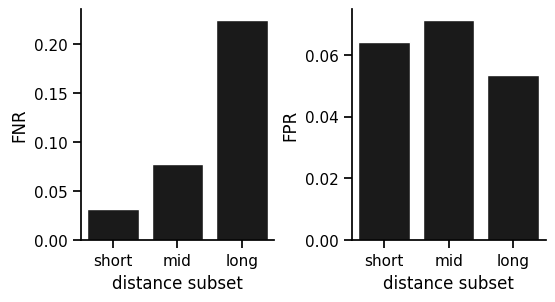## Other visualizations¶

### Distance bias¶

We can visualize and compare the distance bias of different simulations (the degree to which low p-values are enriched or depleted in different distance scales) using the `plot_distance_bias()` function as shown below:

```>>> from hic3defdr import plot_distance_bias
>>>
>>> dist_bins = [
...    ('short', (None, 15)),  # distances in bin units
...    ('mid', (16, 30)),
...    ('long', (31, None))
... ]
>>> _ = plot_distance_bias([h, h_sim], [b for _, b in dist_bins], labels=['real', 'sim'], outfile='images/distance_bias.png')
```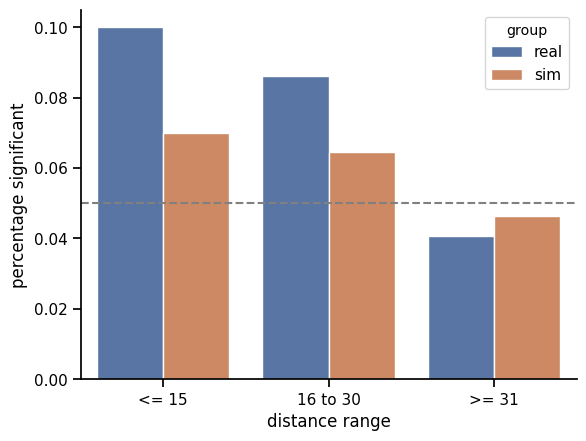## Package structure¶

The `hic3defdr` package is layed out in three parts:

1. `hic3defdr.util`: library of re-usable functions for performing computations related to differential loop calling
2. `hic3defdr.plotting`: library of re-usable functions to plotting visualizations related to differential loop calling
3. `hic3defdr.analysis`: a module that defines the HiC3DeFDR class, which provides an implementation of stitching together all the computational steps and visualizations in an easy-to-use way

The HiC3DeFDR class includes many methods, so to keep things organized these methods are defined in four separate mixin classes which are combined to form the full HiC3DeFDR class in `hic3defdr/analysis/constructor.py`:

• `hic3defdr.analysis.core.CoreHiC3DeFDR`
• `hic3defdr.analysis.analysis.AnalyzingHiC3DeFDR`
• `hic3defdr.analysis.simulation.SimulatingHiC3DeFDR`
• `hic3defdr.analysis.plotting.PlottingHiC3DeFDR`

We recommend that most users simply import the HiC3DeFDR class and interact with this package through that interface, but the functions defined in `hic3efdr.util` and `hic3defdr.plotting` may also be useful to some users and are designed to be somewhat re-usable.

The complete layout of the package is summarized below:

```hic3defdr/                      # package root
├── _version.py                 # version configuration
├── analysis/                   # HiC3DeFDR class and alternatives
│   ├── alternatives.py         # defines alternative analysis models
│   ├── analysis.py             # HiC3DeFDR's pipeline methods
│   ├── constructor.py          # HiC3DeFDR's class definition and constructor
│   ├── core.py                 # HiC3DeFDR's core save/load methods
│   ├── plotting.py             # HiC3DeFDR's plotting methods
│   └── simulation.py           # HiC3DeFDR's simulation/evaluation methods
├── plotting/                   # library of plotting functions
│   ├── dispersion.py           # dispersion/variance fit visualizations
│   ├── distance_bias.py        # distance bias comparison barplots
│   ├── distance_dependence.py  # distance dependence curve comparison
│   ├── fdr.py                  # FDR control curve plotting
│   ├── fn_vs_fp.py             # FN vs FP tradeoff barplots
│   ├── grid.py                 # "pixel detail grid" combination visualization
│   ├── heatmap.py              # simple contact matrix heatmap plotting
│   ├── histograms.py           # p-value/q-value histograms
│   ├── ma.py                   # MA plots
│   └── roc.py                  # ROC curve plots
└── util/                       # library of utility functions
├── binning.py              # creating groups of points
├── balancing.py            # KR matrix balancing
├── banded_matrix.py        # BandedMatrix class (used for filtering)
├── classification.py       # classifying differential loop pixels
├── cluster_table.py        # creating tables summarizing cluster info
├── clusters.py             # interacting with called loop clusters
├── dispersion.py           # estimating dispersions in NB data
├── evaluation.py           # evaluating results of simulations
├── filtering.py            # filtering (applied before balancing)
├── lowess.py               # lowess fitting
├── lrt.py                  # NB likelihood ratio testing
├── matrices.py             # interacting with sparse matrices
├── parallelization.py      # parallelizing work across cores
├── printing.py             # printing updates and info to the console
├── progress.py             # showing progress bars
├── scaled_nb.py            # dealing with scaling factors in NB
├── scaling.py              # scaling reps to account for sequencing depth
├── simulation.py           # simulating pseudoreplicates
└── thresholding.py         # thresholding differential pixels/clusters
```

Additional options are exposed as kwargs on the functions in this library. Use `help(<function>)` to get detailed information about the options available for any function and what these options may be used for.
We run our tests with tox. To execute tests, install tox (`pip install tox`) and then run `tox` to run all tests or `tox -e <testenv>` to run a specific test environment. See `tox.ini` for the full specification of all test environments.